Vignette 18
Continued Fractions

A continued fraction is a fractional expression of the formwhere a0, a1, a2, a3, ... are integers, all positive, with the possible exception of a0. As a convenient shorthand notation, we will denote the continued fraction above by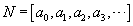.

Finite Continued Fractions

A finite continued fraction -- an expression like that above that actually ends -- represents a rational number.  As an example,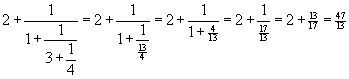What is perhaps more surprising, every rational number can be represented as a finite continued fraction!  To express a given rational number as a continued fraction, we need only perform ordinary division.  For example, to express 221/41 as a continued fraction, we divide 221 by 41 to obtain the quotient 5 and remainder16.  That is,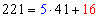.  We continue the division process, each time dividing the most recent divisor by the most recent remainder, stopping only when the remainder is 0: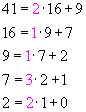This leads to the continued fractionNotice that the numbers 2, 1, 1, 3 and 2 are precisely those colored magenta in the repeated divisions above.

Try this process yourself, with the fraction 23/16.

Infinite Continued Fractions

Infinite continued fractions involve more than ordinary arithmetic, because the chain of fractions never ends.   A formal meaning can be given to an infinite continued fraction in the following way.  The value of the infinite continued fractionis the limit of the sequence of values of the finite continued fractionseach of which makes sense because it is finite.  A somewhat technical argument shows that every infinite continued fraction represents an irrational number.  Moreover, every irrational number can be expressed as an infinite continued fraction!  This fact was proven by the prolific Swiss mathematician Leonhard Euler (1707-1783).  Euler also derived many interesting continued fraction formulas, some of which we will see below.

The Golden Ratio

The most basic of all continued fractions is the one using all 1's:and so a natural question concerns what the value of this continued fraction is.  If we let x denote this value, then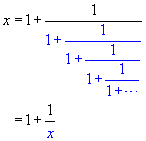In the equation above, notice that the part colored blue is, in fact, identical to x, giving the result that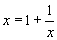.  (Remember that the chain of fractions continues indefinitely, so that the full expression for x is actually embedded within the expression for x !)  Multiplying the equationon both sides by x gives us x2 = x + 1 or, equivalently,.  Thus, the value of the continued fractionmust be a solution to the equation.  Since the value is positive (all of the numbers being added are positive), the quadratic formula gives us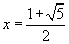, the number known as the golden ratio or golden mean.  This number pops up over and over in unexpected places in mathematics.  The ancient Greeks discovered it in certain geometric constructions, and used it extensively in their architecture.  It occurs repeatedly in nature, as a limiting value of sequences of ratios in certain measurements of flowers and other plant life.  Psychological tests have indicated that the most aesthetically pleasing size for a rectangle is one for which the ratio of length to width is the golden ratio.  The golden ratio appears in art throughout the ages, from Leonardo da Vinci to Piet Mondrian.  So it is certainly fitting that this same number should arise when considering the most basic of continued fractions!

Some Other Continued Fractions

Euler found thatThis is actually easy to see, for if we let x denote the value of this continued fraction, then we haveNoticing that the blue part is identical to x, we see that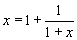.  It is then easy to solve this equation to see that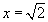.  (Try this yourself.)

Euler also discovered how to write an infinite series as a continued fraction, and vice versa.  Using this technique, he found a number of interesting continued fractions involving the number e -- the number used as the base of the natural logarithm function.  Among the formulas that he discovered are: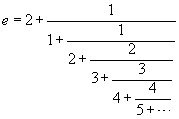and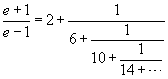Further Exploration

Copyright © 2000 by Carl R. Spitznagel